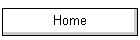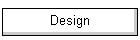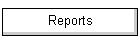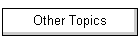One way of trying to understand the amount of downwash produced by a lifting surface is called the "momentum" theory of lift.  In this theory, the lift produced by a wing (fin, rudder, sail) is equal to the downward "push" it gives to the air that it passes through.  By deflecting the air downwards, the wing is lifted upwards.  The following analysis is fairly standard and can be found, for example, in Marchaj's Aerohydrodynamics. The diagram shows a wing "sweeping" through a volume of air in unit time.  The air (or the wing, it is the same thing) moves with a velocity V, and we imagine that as a result of the action of the wing the air is given a downward velocity w in that unit of time.  The force that the wing exerts to make the air change its momentum downwards is the same as the force experienced by the wing in lifting upwards.  The "swept" region of air is somewhat arbitrary, but the theory says it is convenient to imagine this is a cylinder whose diameter is equal to the span of the wing, b.  Before going too much further, it should be obvious that this theory assumes the downwash is constant across the wingspan.  That is, the theory assumes an ideal distribution of downwash, as found with an elliptical wing planform.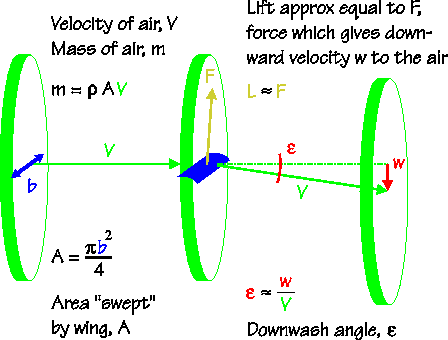We start by saying this force, F, is equal to the mass of the air, m, times its new downward velocity, w, per unit time.  The mass of air is given by its volume times its density, ρ (rho), and the volume in unit time is V times the "swept" area A.  Since we are interested in the downwash angle, ε (epsilon), rather than the actual downwash velocity, we substitute V.ε for w, since for small angles measured in radians (that is, for small w in relation to V), ε is approximately w divided by V.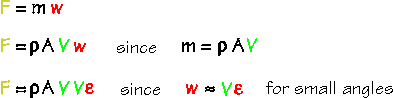Since we are interested in the downwash angle, ε, we re-arrange the formula to find that ε is equal to the force on the wing divided by the changed momentum of the mass of air.  Now the theory does its four clever tricks. (a)  The first is to say that the lift experienced by the wing is approximately equal to the force on the wing due to the air being "pushed" down.  It isn't exactly equal, strictly speaking, because the force of the air being pushed down moves the wing both upwards and rearwards.  This is shown in the diagram later on, where the rearwards action corresponds to drag, but for now we'll use the approximation. (b)  If lift is approximately equal to the force on the wing, we can use our familiar formula for lift, L, (the product of the lift coefficient, the air density ρ, the square of the speed of the air V, and the wing surface area S) in place of the unknown value for the force, F.  Using this formula for lift allows the air density, ρ, to cancel out, as well as the air velocity terms in V. (c)  We calculate the "swept" area, A, being the area of a circle whose diameter is b, the wingspan. (b)  Finally, we note that the aspect ratio of a wing, AR, is defined to be the square of its span divided by its area, A.  This last trick is why the theory started by imaging the "swept" region to be a cylinder.  The span squared term and the wing area term are both taken care of, leaving us with the very simple result, that the downwash angle is the ratio between lift coefficient and aspect ratio, times some constant.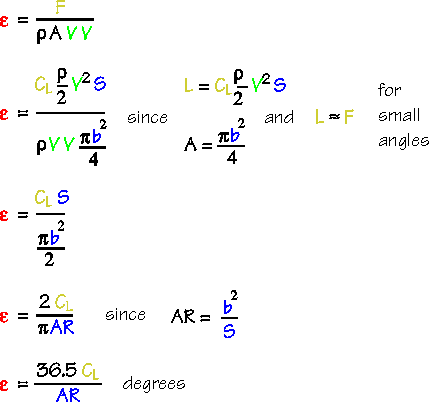Interestingly, downwash is a three-dimensional effect.  It "vanishes" with wings of infinite AR.  This is why textbooks dealing with lift only start to mention downwash when they begin working with "real" wings rather than with the theory of wing sections. If we wish to measure downwash in degrees instead of radians, the constant 2/π is equal to 36.5, and so the downwash angle ε is 36.5 times the lift coefficient divided by the aspect ratio of the wing.  Keep in mind that this result is for a rectangular planform wing.  As the "Downwash" page and spreadsheet shows, increasing wing taper increases the downwash. Now we need to look a little more closely at what is happening in the vicinity of the wing.  Where the downwash angle of the air at the trailing edge of the wing is ε, the total force experienced by the wing is at an angle of half epsilon, ε/2, to the incident flow.  (Do a little experiment in your mind.  Put your hand out of the car window, and deflect some air into the car.  The air would be deflected by 90 degrees, say, if your hand was at an angle of 45 degrees to the incident flow.  And, although you don't really feel it, your hand is being pushed backwards as much as it is being pushed outwards.  The reaction of the lifting surface is at an angle that is half of the angle of deflection.) The total force on the wing is divided into two parts.  There is a lifting force, normal to the incident flow, and a drag force, acting along the direction of flow.  By trigonometry, the lift component is F times cos (ε/2), and the drag component is F times sin (ε/2). Because the wing has deflected the air and produced some lift, it has also therefore produced some drag.  This drag is called "induced" drag, induced by the fact that lift has been developed.  And the angle by which the total wing force has been rotated backwards, ε/2, is called the "induced" angle, αi.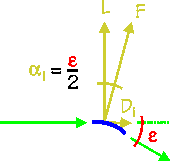In keeping with the idea that the wing reaction angle is half the downwash angle, we can say that the air flowing past the wing, in the region between the leading and trailing edges, is "half way" to being fully deflected.  So the wing is "flying" in air that has already been deflected by the "induced" angle, αi.  We are accustomed to saying that the wing (sail, fin, rudder) makes an angle of attack to the incident flow, alpha, α, but this theory now says that only part of that angle of attack is really "effective".  The other part, αi, can't be involved in lift production because the wind has already been deflected by that amount.  The consequence of this result is to say that, for a given lift coefficient, the angle of attack needs to be higher if the wing has a low aspect ratio.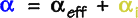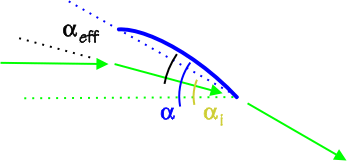The part of the angle of attack that is ineffective, the induced angle, is half the downwash angle.  In degrees, this is 18.24 times the lift coefficient divided by the aspect ratio.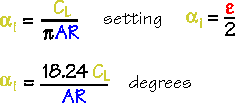To find out the induced drag due to lift, we can use our earlier finding about the value of the downwash angle.  It turns out that the induced drag coefficient is the ratio of the square of the lift coefficient to the aspect ratio, times some constant.  Induced drag starts out as quite a small value, and then increases rapidly as lift increases.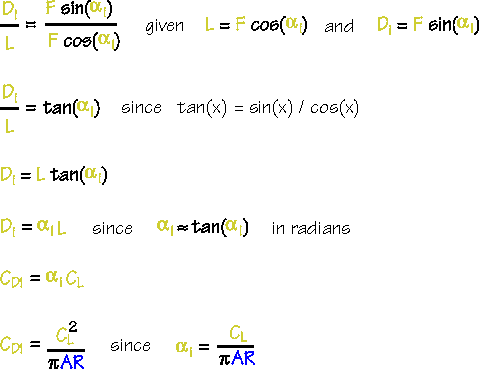2011-07-13